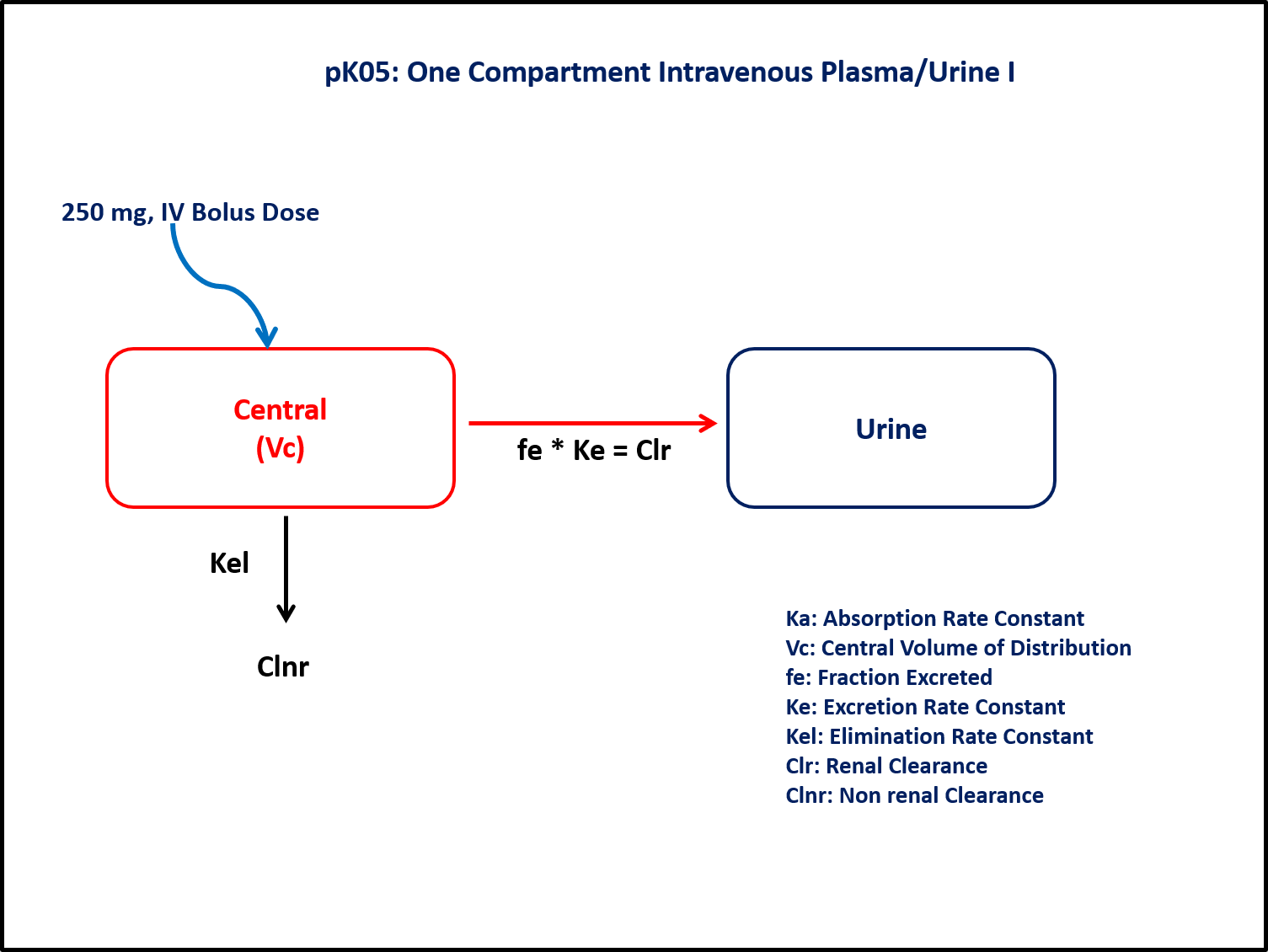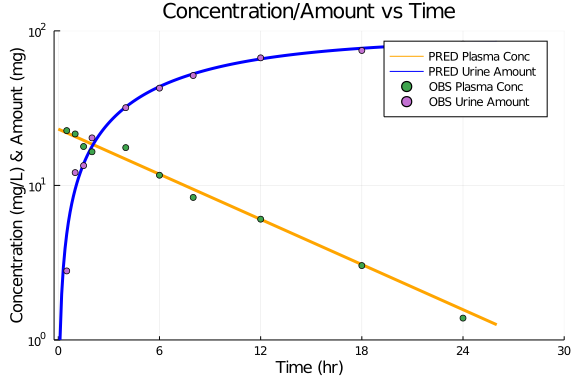# Exercise PK05 - One Compartment Intravenous/Urine 1

### Background

• Structural Model - One Compartment Model with urinary excretion

• Route of Administration - Intravenous Bolus

• Dosage Regimen - 250mg IV Bolus

• Subject - 1### Learning Outcome

In this model,both plasma and urine data is collected and will help to estimate parameters like Clearance, Volume of Distribution and fraction of dose excreted in urine.

### Objectives

In this tutorial, you will learn to build a one compartment model with urinary excretion and simulate the model for one single subject and single dosage regimen

### Libraries

call the "necessary" libraries to get started.

using Pumas
using Plots
using CSV
using StatsPlots
using Random


### Model

In this one compartment model, we administer an IV dose in central compartment.

pk_05         = @model begin
@param begin
tvVc      ∈ RealDomain(lower=0)
tvClr     ∈ RealDomain(lower=0)
tvClnr    ∈ RealDomain(lower=0)
Ω         ∈ PDiagDomain(3)
σ²_prop   ∈ RealDomain(lower=0)
end

@random begin
η         ~ MvNormal(Ω)
end

@pre begin
Clr       = tvClr * exp(η)
Clnr      = tvClnr * exp(η)
Vc        = tvVc * exp(η)
end

@dynamics begin
Central'  = -(Clnr/Vc)*Central - (Clr/Vc)*Central
Urine'    =  (Clr/Vc)*Central
end

@derived begin
cp_plasma = @. Central/Vc
dp_plasma ~ @. Normal(cp_plasma, sqrt(cp_plasma^2*σ²_prop))
cp_urine  = @. Urine
end
end

PumasModel
Parameters: tvVc, tvClr, tvClnr, Ω, σ²_prop, σ_add
Random effects: η
Covariates:
Dynamical variables: Central, Urine
Derived: cp_plasma, dp_plasma, cp_urine, dp_urine
Observed: cp_plasma, dp_plasma, cp_urine, dp_urine


### Parameters

• Clnr - Non renal Clearance(L/hr)

• Clr - Renal Clearnace(L/hr)

• Vc - Volume of the Central Compartment(L)

• Ω - Between subject variability

• σ - Residual Error

param = (tvVc    = 10.7965,
tvClr   = 0.430905,
tvClnr  = 0.779591,
Ω       = Diagonal([0.0,0.0,0.0]),
σ²_prop = 0.015,

(tvVc = 10.7965, tvClr = 0.430905, tvClnr = 0.779591, Ω = [0.0 0.0 0.0; 0.0
0.0 0.0; 0.0 0.0 0.0], σ²_prop = 0.015, σ_add = 3)


### Dosage Regimen

A single dose of 250mg given as an Intravenous bolus to single subject.

ev1  = DosageRegimen(250, time=0, cmt=1)
sub1 = Subject(id=1, events=ev1)

Subject
ID: 1
Events: 1


### Simulation

Lets simulate the plasma concentration and unchanged amount excreted in urine.

Random.seed!(123)
sim_sub1 = simobs(pk_05,sub1,param,obstimes=0:0.1:26)
df1      = DataFrame(sim_sub1)


### Dataframe and Plot

df_plot = filter(x -> x.time in [0.5,1,1.5,2,4,6,8,12,18,24], df1)
@df df1 plot(:time, :cp_plasma, yaxis=:log,
label="PRED Plasma Conc", xlabel="Time (hr)", ylabel="Concentration (mg/L) & Amount (mg)",
title = "Concentration/Amount vs Time",
color=[:orange], linestyle=[:solid], linewidth=3,
ylims=(1,100), xlims=(-0.25,30), xticks=[0,6,12,18,24,30], yticks=[1,10,100])
@df df1 plot!(:time, :cp_urine, yaxis=:log,
label="PRED Urine Amount",color=[:blue], linestyle=[:solid], linewidth=3)
@df df_plot scatter!(:time, :dp_plasma, yaxis=:log,
label="OBS Plasma Conc")
@df df_plot scatter!(:time, :dp_urine, yaxis=:log,
label="OBS Urine Amount")